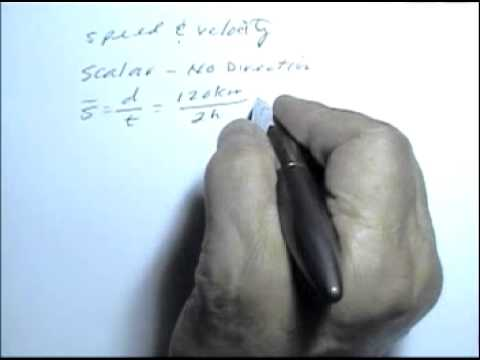### Main Difference

Speed and velocity are the two interrelated terms that are often used interchangeably, but the one knowing basics of physics can easily differentiate it between both these terms. While differentiating it between both these terms, informally, we can say that velocity is the speed with a direction. Actually, the speed is the magnitude of velocity which is irrespective of direction. Therefore, speed is a scalar quantity (needs no direction for representation), whereas velocity is the vector quantity (needs direction for representation). For example, when we say that we are driving at 80km/h, this will be the speed, although when we will say that we are driving at 80km/h towards East, this is the velocity. Speed is the distance traveled by a body within the specific time, on the other hand, velocity is the rate of change of displacement of the body within the specific time and in a specific direction. The speed of the object can be calculated as distance traveled /time taken, while the velocity of an object can be calculated using formula displacement/time taken.

### Comparison Chart

 Speed Velocity Definition Speed is the distance traveled by a body within the specific time. Velocity is the rate of change of displacement of the body within the specific time and in a specific direction. Type of Quantity Scalar Quantity Vector Quantity Formula distance traveled /time taken displacement/time taken Example When we say that we are driving at 80km/h, this will be the speed. When we will say that we are driving at 80km/h towards East, this will be the velocity.

### What is Speed?

Speed is the distance covered by a body in per unit time irrespective of the direction. It is a scalar quantity as it doesn’t needs direction for its representation. The speed of the object can be simply calculated by using the formula distance traveled /time taken. Some of the other famous concepts of speed are instantaneous speed and average speed, one often mix these terms. When we are travelling on a car at speed of 100km/h, we say that we are going at speed of 100km/h, actually this is the instantaneous speed, which means it would be the speed of the body at that specific time , it may vary at any other time. Although when we refer to the average speed, it is the total distance covered/ total time taken. For example, you have covered 400 km’s of distance in 5 hours, the average speed will be 80km/h (diving total distance by the total time taken). The instantaneous speed within the 5 hours of traveling might have fallen or increase, but the most likely speed carried on with comes as the average speed f the object. The SI unit of measuring speed is ms-1, although it can also be denoted in miles per hour or kilometer per hour depending upon the distance being covered with respect to time.

### What is Velocity?

Velocity is the rate of change of displacement of the body in per unit time in the specific direction.. t is the vector quantity as it needs direction as compulsory for its representation. Actually the reason of speed being scalar and velocity being vector quantity is that, speed deals with the distance, which itself is a scalar quantity, whereas velocity deals with the displacement, which itself is a vector quantity. Displacement can be defined as the change of position of the body. The most relative term to velocity is the acceleration, which is actually the change of velocity of the body. When the body in motion changes its velocity, actually it is accelerating. As mentioned above velocity needs direction for its representation, when we say that we are travelling at 60mph towards North, it is the velocity and here if we will not discuss the direction ‘North’, it will be the speed. The SI unit of velocity is the same as for the speed, ms-1.

### Speed vs. Velocity

• Speed is the distance traveled by a body within the specific time, on the other hand, velocity is the rate of change of displacement of the body within the specific time and in a specific direction.
• Speed is a scalar quantity (needs no direction for representation), whereas velocity is the vector quantity (needs direction for representation).
• The speed of the object can be calculated as distance traveled /time taken, while the velocity of an object can be calculated using formula displacement/time taken.